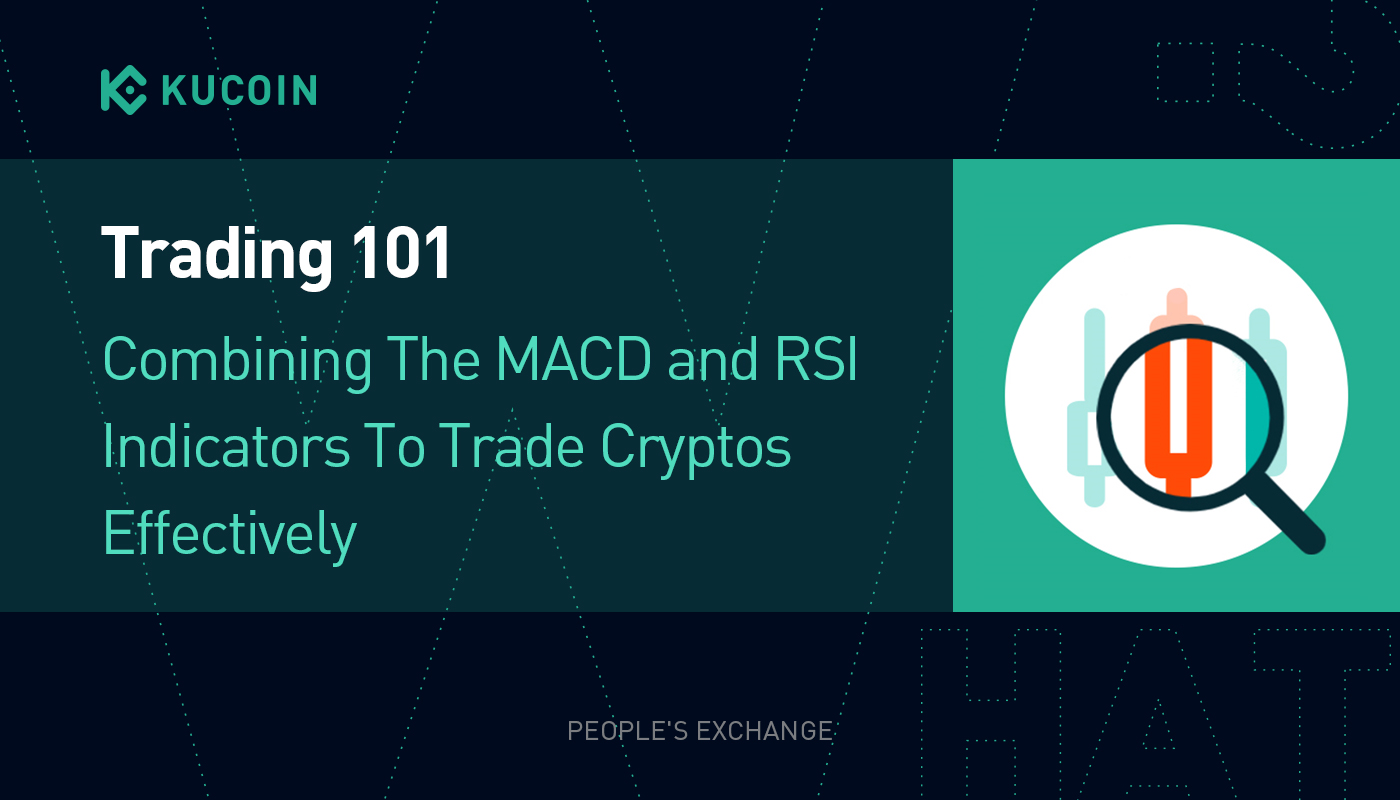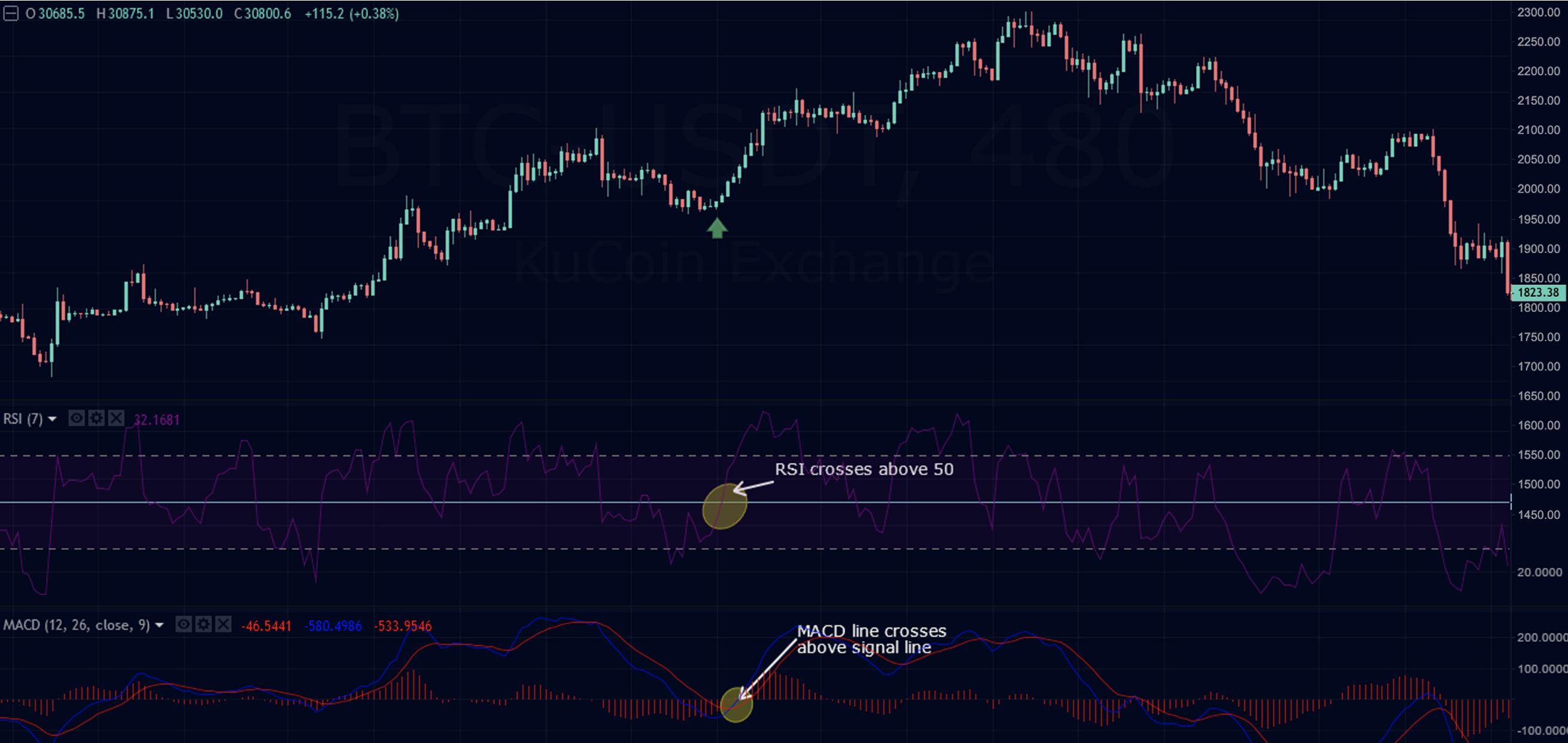Details# Trading 101: Combining The MACD and RSI Indicators To Trade Cryptos Effectively

2021/08/19 09:21:31

With the volatile nature of the crypto market, it is prudent to trade using momentum and trend technical indicators. The success rate of trade is likely to increase by combining both types of indicators to show the magnitude and direction of the price action.

A typical mistake most traders make is the assumption that the knowledge of the market trend is enough to guarantee profitability. Momentum indicators should be part of any trading strategy, if possible, as they provide you with information about the trend’s strength. You can also use these indicators to identify possible reversals, i.e., when the trend loses strength.

The indicator values usually oscillate around a baseline within a predefined range, but their oscillation may not be limited within a range. Because momentum measures the rate of change, a rapid price increase leads to strong momentum, while slower price changes indicate weak momentum.

This is where the MACD and RSI indicators come in.## Moving Average Convergence Divergence (MACD)

MACD is a trend indicator. The construction of the MACD indicator is based on the difference between two exponential moving averages (EMAs).

With the default settings of the MACD, you can create the MACD line and the signal line.

MACD Line = 12-Day Simple Moving Average (EMA) – 26-Day Simple Moving Average (EMA)

Signal Line = 9 Days Single Moving Average (EMA) of the MACD Line

Of course, with the advent of the internet and technology, all these calculations occur in the back-end of your trading platform. Here are the rules for trading MACD.

MACD settings

### Use the following MACD default settings:

• Fast EMA:12
• Slow EMA: 26
• Smoothed: 9 (signal line)

• The MACD crosses above the signal line.
• The MACD histogram crosses above the zero line.
• Go long when the MACD crosses over its signal line, but only when the subsequent candle is bullish.

• The MACD crosses below the signal line.
• The MACD histogram crosses below the zero line.
• Sell when the MACD crosses below its signal line, but only when the subsequent candle is bearish.

## The Relative Strength Index (RSI)

As the name suggests, the RSI is used to determine the momentum (i.e., strength) of a trend. The indicator is made up of a line that oscillates between 0 and 100. Typically, when the RSI is above 70, the market is regarded as overbought, but it is said to be oversold when it’s below 30.

The RSI captures the strength of price movement relative to time and the momentum of the rise or fall of a market movement. The Relative Strength Index provides information about whether a market is in the overbought zone or the oversold zone.

• A market is defined as ‘overbought’ when it is above 70 on the scale of values, which means that an end to an uptrend would be expected.
• On the other hand, a market is defined as ‘oversold’ if it is below 30 on the scale of values, which means that an end to a downward trend would be expected.
• A value above 50 indicates that the strength of the buyers predominates, so prices rise; a value below 50 indicates that the sellers dominate and that prices fall accordingly.

The RSI indicator has a value scale from 0 to 100 and refers to the ratio of an average price increase to an average price decline. These averages are calculated like the exponential moving average before the relative strength is converted into an index that fluctuates between 0 and 100.

It is important to note that the RSI is both a lagging indicator and a leading indicator. It is widely used by traders in combination with other indicators and oscillators to generate accurate trading signals.

## Double Confirmation Momentum Strategy Using RSI and MACD Indicators

Traders can use both the RSI and the MACD to set up a trade. When combining RSI and MACD, one waits for both indicators to give a trading signal. Here’s the general rule of thumb when using this trading strategy. The RSI gives a trading signal when the RSI line is moved away from the overbought or oversold area. The MACD gives the trading signal when the short-term MACD line intersects the long-term signal line.

The position is closed as soon as the RSI returns to an extreme range and the MACD lines cross each other again.

With the RSI and MACD, the buy signal is generated when:

• The RSI has crossed above 50. It shows that the bullish momentum is picking up.
• The MACD histogram crosses above the 0-level, and the MACD line crosses above the signal line.
• Close the position when the RSI reaches 70.

As you can see in the Ethereum (ETH) price-chart, all the rules we discussed above were respected by the market and we can see a favorable market movement.Double Confirmation Buy-Signal Example on the Ethereum Price Chart | Source: ETH/USDT

### Sell Signal

With the RSI and MACD, the sell signal is generated when:

• The RSI has crossed below 50. It shows that the bearish momentum is picking up.
• The MACD histogram crosses below the 0-level, and the MACD line crosses below the signal line.
• Close the position when the RSI reaches 30.

From the below Bitcoin price chart, we can see the RSI line dropping below 50 while the histograms in MACD dropped below zero at the same time. Also, we can see the MACD line crossing below the signal line which is a clear sell signal.Double Confirmation Sell-Signal Example on the Bitcoin Price Chart | Source: BTC/USDT

## Conclusion

Combining the MACD and the RSI indicators is the simplest and the most effective way of trading with market trends and momentum. However, as is the case with any other trading strategy, often use it with candlestick chart patterns. This will help you avoid any potential false signals generated by the double confirmation. We hope you find this article informative. Keep following the KuCoin blog for more interesting educational content. All the best!